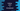# Python program to print a full pyramid## Python program to print a full pyramid:

Pattern printing programs are the best ways to practice a programming language, and these are the most commonly asked questions in programming tests.

In this post, I will show you how to print a full pyramid in python.

A full pyramid looks as like below:

``````    *
* *
* * *
* * * *
* * * * *``````

This is a full pyramid of size 5.

We can print a pattern like this of any size. This program will take the height of the pyramid as input from the user and then it will print it using *. You can use any other character if you want.

### Algorithm behind printing a full pyramid:

To understand the algorithm behind the program we will use below, let me show you a different pattern:

``````yyyy*
yyy* *
yy* * *
y* * * *
* * * * *``````

This is similar to the above one. If you replace all y with blank space, it will be the above pyramid.

So, let’s try to write the algorithm for this pyramid. Let’s say row numbers of this pyramid are from 0 to 4:

• For row 0, we are printing 4 y and one *
• For row 1, we are printing 3 y and two *
• For row 2, we are printing 2 y and three * etc.

That means, for row n, we are printing height - n - 1 number of y and n + 1 number of *. Also, we need one blank space at the end of each *.

If we replace y with blank, it will print the required triangle.

From the above observation, we can conclude the algorithm:

• Run one for loop from 0 to height - 1
• Inside the loop, print blank for height - i - 1 number of times and print * for i + 1 number of times, where i is the variable used in the for loop.
• Print a new line at the end of each row.

### Python program:

Below is the complete python program that prints this pattern:

``````height = int(input('Enter the height : '))

for i in range(height):
for j in range(height - i - 1):
print(' ', end='')
for j in range(i+1):
print('*', end=' ')
print('\n')``````

If you run this program, it will print output as like below:

``````Enter the height : 5
*

* *

* * *

* * * *

* * * * *

Enter the height : 10
*

* *

* * *

* * * *

* * * * *

* * * * * *

* * * * * * *

* * * * * * * *

* * * * * * * * *

* * * * * * * * * * ``````